Courses

15 Questions MCQ Test Quantitative Aptitude (Quant) | Test: Quadratic Equations- 4

Description
Attempt Test: Quadratic Equations- 4 | 15 questions in 15 minutes | Mock test for CAT preparation | Free important questions MCQ to study Quantitative Aptitude (Quant) for CAT Exam | Download free PDF with solutions
QUESTION: 1

Find the maximum value of the expression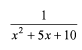Solution:

For the given expression to be a maximum, the denominator should be minimized. (Since, the function in the denominator has imaginary roots and is always positive), x2 + 5x + 10 will be minimized at x = -2.5 and its minimum values at x = -2.5 is 3.75.
Hence, required answer = 1/3.75 =4/15.

QUESTION: 2

Find the minimum value of the expression (p +1/p); p > 0.

Solution:

The minimum value of (p + 1/p) is at p = 1. The value is 2.

QUESTION: 3

The expression a2 + ab + b2 is _________for a < 0, b < 0

Solution:

For a, b negative the given expression will always be positive since, a2, b2 and ab are all positive.

QUESTION: 4

Find the roots of the quadratic equation: x2 + 2x - 15 = 0?​

Solution:

x2 + 5x - 3x - 15 = 0
x(x + 5) - 3(x + 5) = 0
(x - 3)(x + 5) = 0
=> x = 3 or x = -5.

QUESTION: 5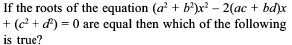Solution:

Solve this by assuming each option to be true and then check whether the given expression has equal roots for the option under check.

Thus, if we check for option (b). ad = be.
We assume a = 6, d = 4 b = 12 c = 2 (any set o f values that satisfies ad = bc).
Then (a2 + b2) x2 - 2(ac + bc) x + (c2 + d2) = 0

180 x2- 120 x +20 = 0.
We can see that this has equal roots. Thus, option (b) is a possible answer. The same way if we check for a, e and d we see that none of them gives us equal roots and can be rejected.

QUESTION: 6

Two numbers a and b are such that the quadratic equation ax2 + 3x + 2b = 0 has - 6 as the sum and the product of the roots. Find a + b.

Solution: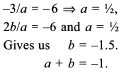QUESTION: 7

Find the value of the expression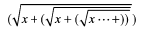Solution: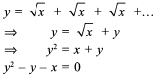Solving quadratically, we have option (b) as the root of this equation.

QUESTION: 8

If the roots of the equation (a2 + b2) x2 - 2b(a + c) x + (b2 + c2) = 0 are equal then a, b, c, are in

Solution:

Solve by assuming values of a, b, and c in AP, GP and HP to check which satisfies the condition.

QUESTION: 9

If x2 + ax + b leaves the same remainder 5 when divided by x - 1 or x + 1 then the values of a and b are respectively

Solution: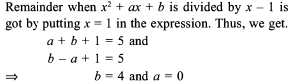QUESTION: 10

Read the data given below and it solve the questions based on.
If α and β? are roots of the equation x2 + x - 7 = 0 then.

Find α2 + β2

Solution: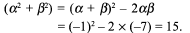QUESTION: 11

For what values o f c in the equation 2x2 - (c3 + 8c - l)x + c2 - 4c = 0 the roots of the equation would be opposite in signs?

Solution:

For the roots to be opposite in sign, the product should be negative.

(c2 - 4x)/2 < 0 ⇒ 0 < c < 4 .

QUESTION: 12

The expression x2 + kx + 9 becomes positive for what values of k (given that x is real)?

Solution:

The roots should be imaginary for the expression to be positive i.e.

k2 - 36 < 0

thus - 6 < k < 6 or |k| < 6.

QUESTION: 13

In the Maths Olympiad of 2020 at Animal Planet, two representatives from the donkey’s side, while solving a quadratic equation, committed the following mistakes: (i) One of them made a mistake in the constant term and got the roots as 5 and 9. (ii) Another one committed an error in the coefficient of x and he got the roots as 12 and 4.But in the meantime, they realised that they are wrong and they managed to get it right jointly. Find the quadratic equation.

Solution:

From (i) we have sum o f roots = 1 4 and from (ii) we have product of roots = 48.
Option (c) is correct.

QUESTION: 14

For what value of b and c would the equation x2 + bx + c = 0 have roots equal to b and c.

Solution:

Solve using options. It can be seen that at b = 0 and c = 0 the condition is satisfied. It is also satisfied at b = 1 and c = - 2 .

QUESTION: 15

A journey between Mumbai and Pune (192 km apart) takes two hours less by a car than by a truck. Determine the average speed of the car if the average speed of the truck is 16 km/h less than the car.

Solution:

Solve using options, If the car’s speed is 48 kmph, the bus’s speed would be 32 kmph. The car would take 4 hours and the bus 6 hours.A journey between Mumbai and Pune (192 km apart) takes two hours less by a car than by a truck. Determine the average speed of the car if the average speed of the truck is 16 km/h less than the car. (a) 48 km/h (b) 64 km/h (c) 16 km/h (d) 24 km/hUse Code STAYHOME200 and get INR 200 additional OFF Use Coupon Code

How to Prepare for CAT

Read our guide to prepare for CAT which is created by Toppers & the best Teachers

Track your progress, build streaks, highlight & save important lessons and more!

Similar ContentRelated tests